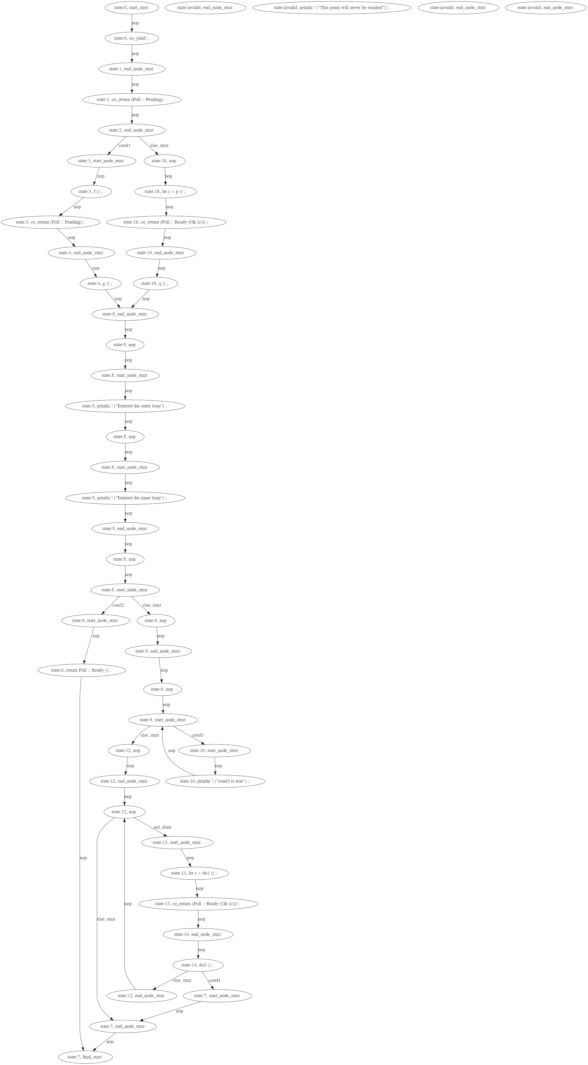## macro gentian

gentian: a proc macro that transforms generators to state machines

### 8 releases

 0.1.8 Apr 1, 2022 Mar 24, 2022 Feb 24, 2022

#656 in Asynchronous

53KB
1K SLoC

# gentian

This crate provides a proof-of-concept proc macro attribute that allows transforming generators to state machines. This crate will be used as an auxiliary tool for v2ray-rust.

## Motivation

Rust's `async` and `await` are cool. But when you manually implement `Future`/`Stream`/`Poll`, the problem comes. Either give up a certain performance to extend the lifetime of the `future` and `poll` it or manually maintain the state machine. When the logic of `poll` is gradually complicated, the correctness of the state machine becomes more difficult to guarantee. Unstable Rust's standard library provides `generator` but it is not suitable for solving the above problems. In summary, this crate is to support using `yield/yield return` in a subset of rust's control flow. And, same as `async` and `await`, it will be compiled into state machines.

## Example

``````use gentian::gentian;

#[cfg(test)]
struct MyGenerator {
my_state_1: usize,
pub my_state_2: usize,
pub num: u32,
pub num1: u32,
}

#[cfg(test)]
impl MyGenerator {
pub fn new() -> MyGenerator {
MyGenerator {
my_state_1: 0,
my_state_2: 0,
num: 0,
num1: 0,
}
}

#[gentian]
#[gentian_attr(state=self.my_state_1)]
pub fn test_simple(&mut self) {
loop {
println!("Hello, ");
//co_yield;
while self.num1 < 99 {
println!("Generator{}", self.num1);
self.num1 += 1;
co_yield;
}
return;
}
}

// state_name , return_default_value
#[gentian]
#[gentian_attr(state=self.my_state_2,ret_val=0u32)]
pub fn get_odd(&mut self) -> u32 {
loop {
if self.num % 2 == 1 {
co_yield(self.num);
}
self.num += 1;
}
}
}

#[test]
fn test_generator_proc_macro() {
let mut gen = MyGenerator::new();
gen.test_simple(); // print Hello,
for _ in 0..200 {
gen.test_simple(); // only print 99 times `Generator`
}
gen.test_simple(); // print nothing
assert_eq!(gen.num1, 99);
for i in (1u32..1000).step_by(2) {
assert_eq!(gen.get_odd(), i);
}
}

``````

## Explanation

The following code is not a valid rust function but showing the logic of generating code.

``````pub fn poll_read_decrypted<R>(
&mut self,
ctx: &mut Context<'_>,
r: &mut R,
dst: &mut [u8],
) -> Poll<io::Result<(usize)>>
where
{
co_yield;
co_return(Poll::Pending);
if cond1{
f();
co_return(Poll::Pending);
g();
}else{
let c=p();
q();
}
'outer: loop {
println!("Entered the outer loop");

'inner: loop {
println!("Entered the inner loop");

// This would break only the inner loop
//break;

// This breaks the outer loop
break 'outer;
}

println!("This point will never be reached");
}
loop{
if cond2{
} else{
break;
}
}
loop{
if cond3{
println!("cond3 is true");
continue;
} else{
break;
}
}
while not_done{
let c=do1();
do2();
if cond4{
break;
}
}
}
``````

The above code would be expanded as:

``````pub fn poll_read_decrypted<R>(
&mut self,
ctx: &mut Context<'_>,
r: &mut R,
dst: &mut [u8],
) -> Poll<io::Result<(usize)>>
where
{
'genloop: loop {
match state {
50 => {
break 'genloop;
}
0 => {
state = 1;
return;
}
1 => {
state = 2;
return Poll::Pending;
}
2 => {
state = 18;
if cond1 {
state = 3;
continue 'genloop;
}
}
3 => {
f();
state = 4;
return Poll::Pending;
}
4 => {
g();
state = 5;
}
5 => {
println!("Entered the outer loop");
println!("Entered the inner loop");
state = 9;
if cond2 {
state = 6;
continue 'genloop;
}
}
6 => {
state = 7;
}
7 => {
state = 50;
}
9 => {
state = 12;
if cond3 {
state = 10;
continue 'genloop;
}
}
10 => {
println!("cond3 is true");
state = 9;
}
12 => {
state = 7;
if not_done {
state = 13;
continue 'genloop;
}
}
13 => {
let c = do1();
state = 14;
}
14 => {
do2();
state = 12;
if cond4 {
state = 7;
continue 'genloop;
}
}
18 => {
let c = p();
state = 19;
}
19 => {
q();
state = 5;
}
_ => {
break 'genloop;
}
}
}
return Poll::Pending;
}
``````

The CFG (control flow graph) of above code is~2MB
~40K SLoC